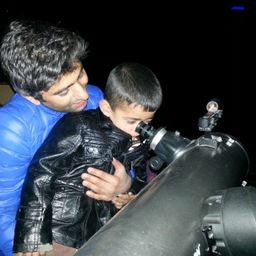Gravitation

### Discussion

You must be signed in to discuss.
##### Top Physics 101 Mechanics Educators##### Christina K.

Rutgers, The State University of New Jersey

LBLectures

Join Bootcamp

### Video Transcript

Well, we know date M C Square is equal to captain G times a moss off son Multiply by small M divided by r squared and solving for our our is equal to G times muscle son divided by C Square. Let's plug in the values well. The gravitational constant is 6.67 multiplied by 10 to the power minus 11. Multiply very massive son, which is 1.99 Multiply by, uh, 10 to the bomber 30. Divided by r and R in this case is to multiply by but to multiply by. Sorry, huh? Three. It's tree multiply guy. It's not our rather it, sir. Speed of light. Excuse me. It's speed off Light and speed of light is three Multiply by 10 to the power eight whole square and therefore are is equal to 1470 meters#### Topics

Gravitation

##### Top Physics 101 Mechanics Educators##### Christina K.

Rutgers, The State University of New Jersey

LBLectures

Join Bootcamp